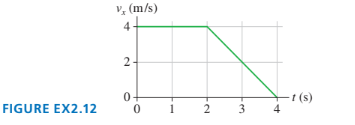Problem: FIGURE EX2.12 shows the velocity-versus-time graph for a particle moving along the x-axis. Its initial position is x0 = 2.0 m at t 0 = 0 s.(a) What are the particle’s position, velocity, and acceleration at t = 1.0 s?(b) What are the particle’s position, velocity, and acceleration at t = 3.0 s?

FREE Expert Solution
93% (314 ratings)
Problem Details

FIGURE EX2.12 shows the velocity-versus-time graph for a particle moving along the x-axis. Its initial position is x0 = 2.0 m at t 0 = 0 s.
(a) What are the particle’s position, velocity, and acceleration at t = 1.0 s?
(b) What are the particle’s position, velocity, and acceleration at t = 3.0 s?